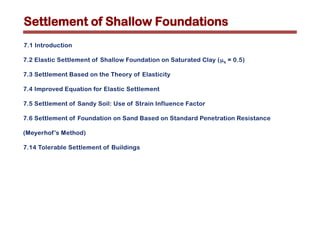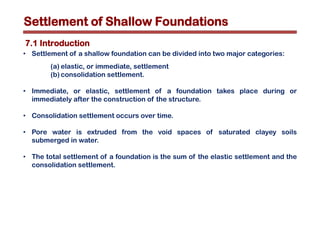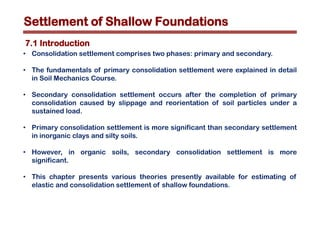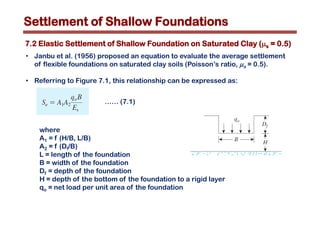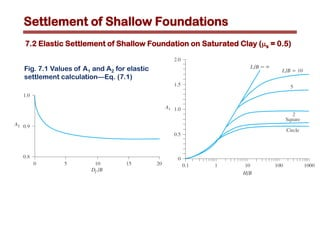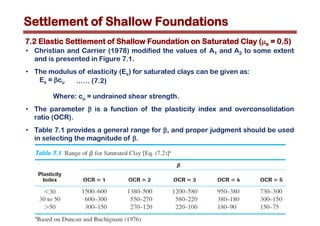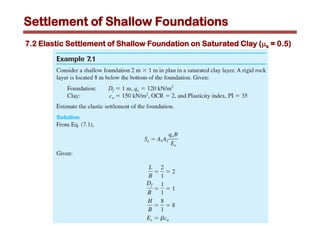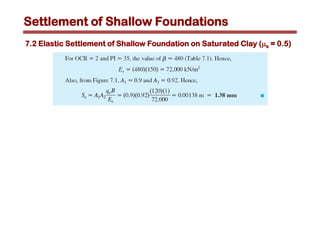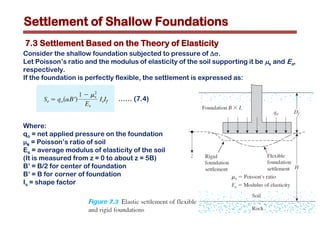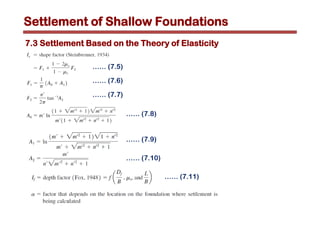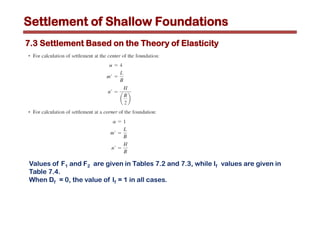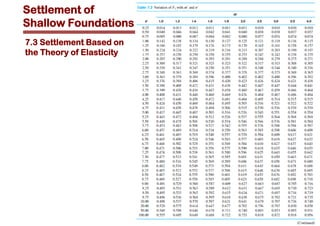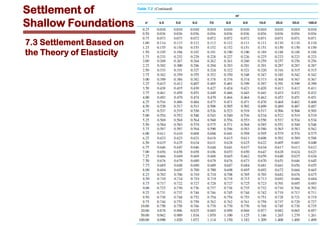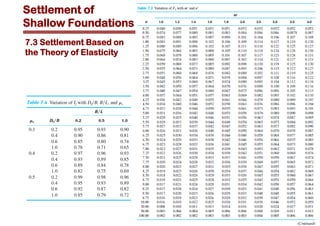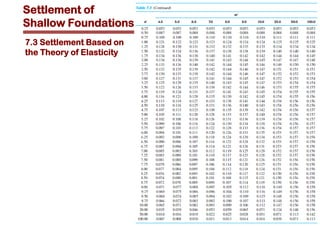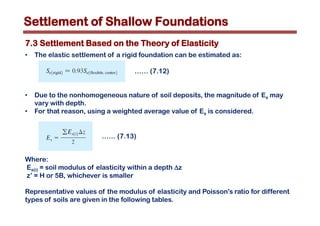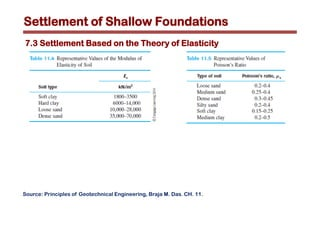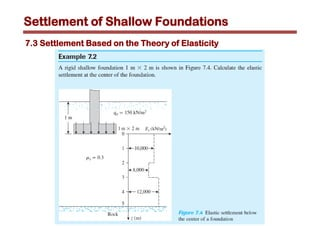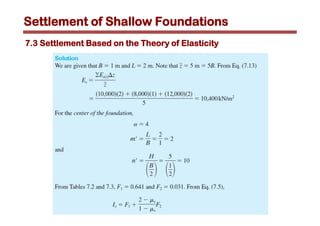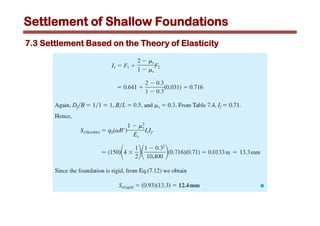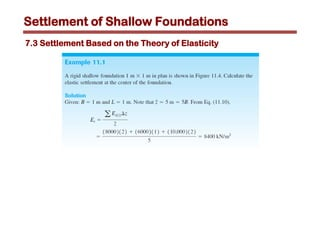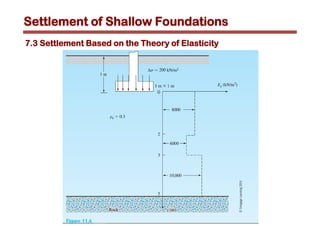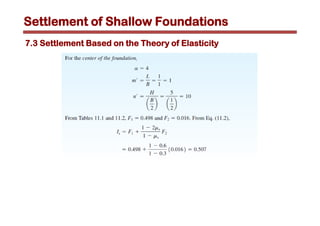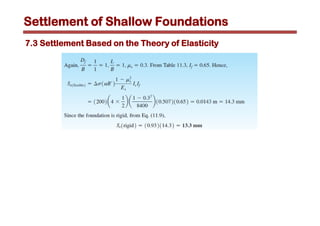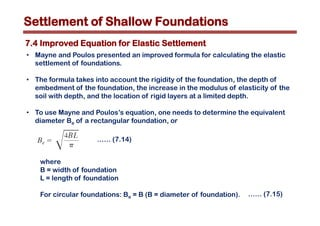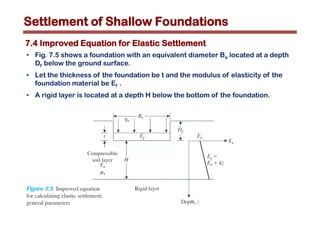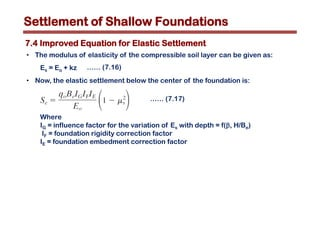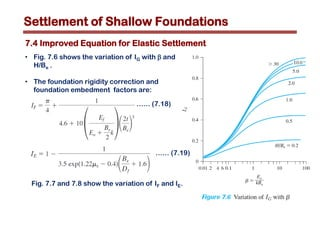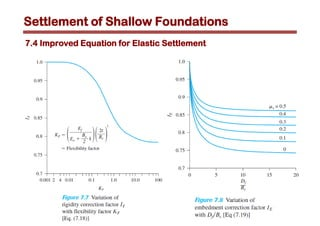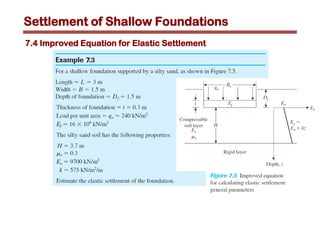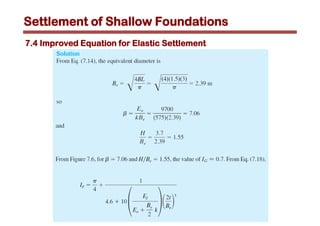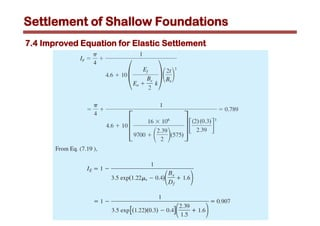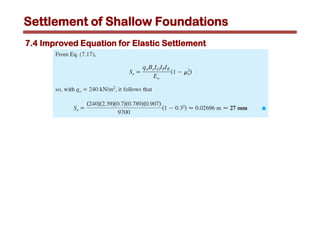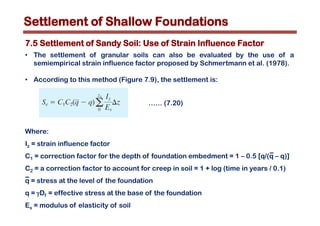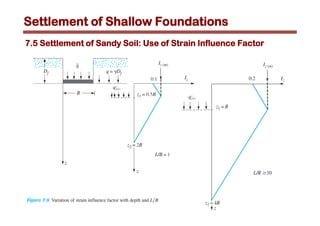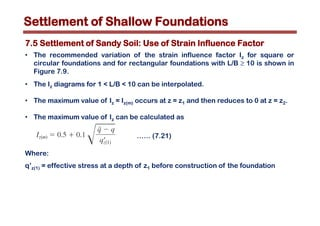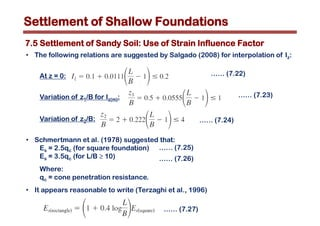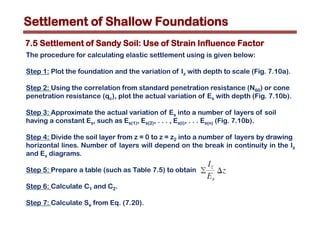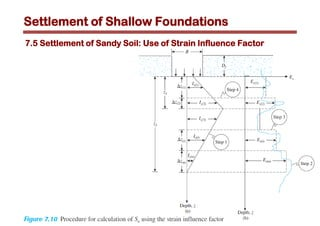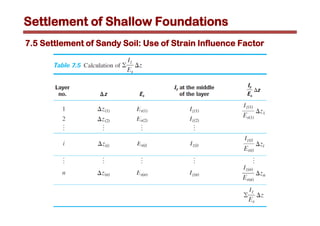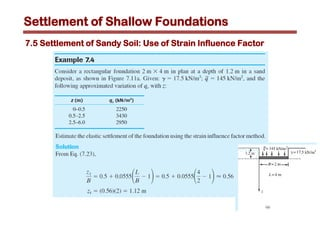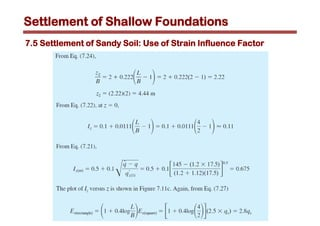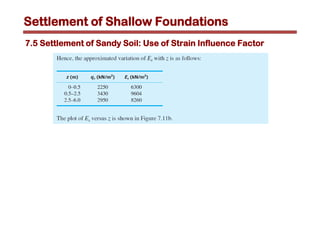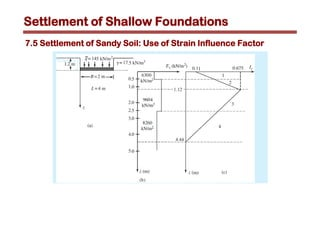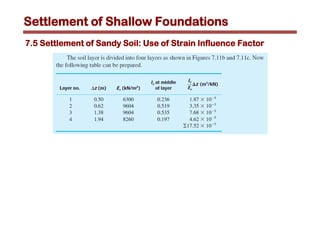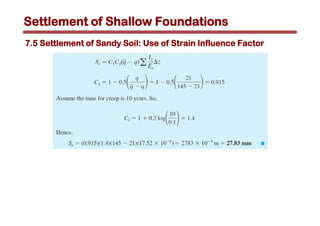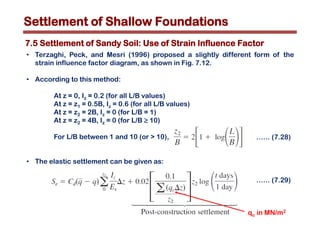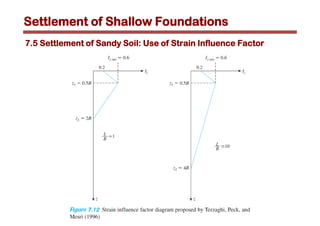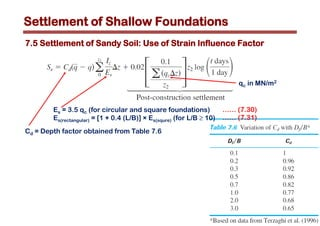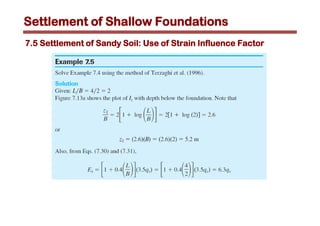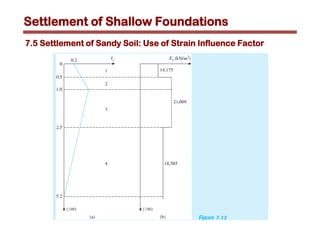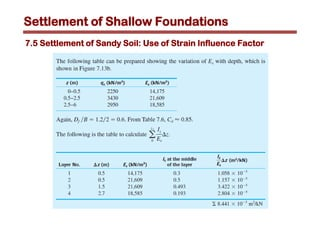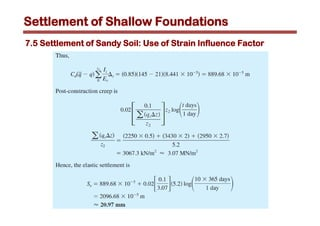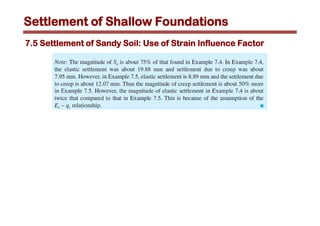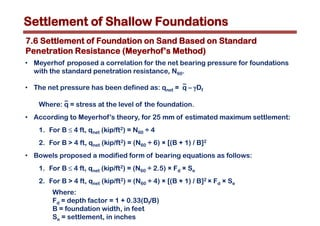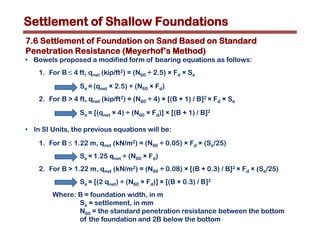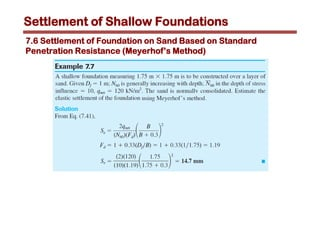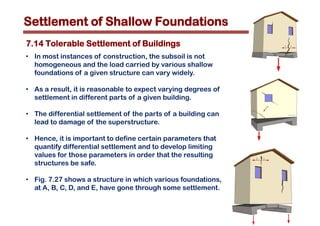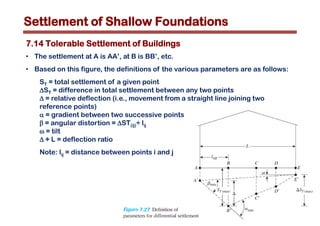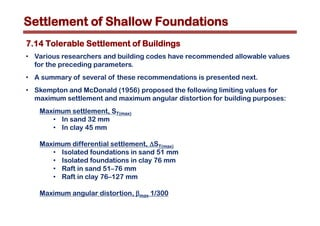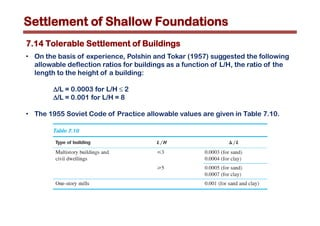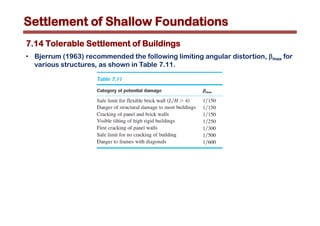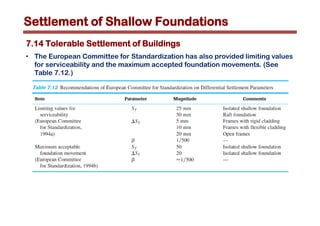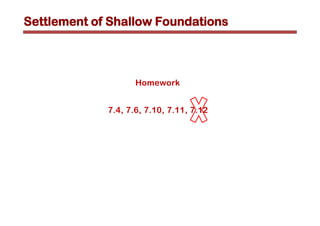1 of 65

## RecommendedUnit7 skpPavan Kumar NCh9 settlement (1)Tharach JanesuapasaereeEffect of foundation flexibility on dynamic behaviour of asymmetric building ...eSAT JournalsChapter 4. Bearing Capacity of Soil.pdfgashutube3RD sem progress of thesis MINAR.pptxMinarIslam2Lecture17Wildcat23

### Chapter 7.pdf

1. Foundation Engineering Engineering Department Building Technology (Civil) Engineering Instructor: Luay I. Qrenawi, PhD Candidate Second Semester 2018 – 2019 1
2. 7.1 Introduction 7.2 Elastic Settlement of Shallow Foundation on Saturated Clay (ms = 0.5) 7.3 Settlement Based on the Theory of Elasticity 7.4 Improved Equation for Elastic Settlement 7.5 Settlement of Sandy Soil: Use of Strain Influence Factor 7.6 Settlement of Foundation on Sand Based on Standard Penetration Resistance (Meyerhof’s Method) 7.14 Tolerable Settlement of Buildings Settlement of Shallow Foundations
3. Settlement of Shallow Foundations • Settlement of a shallow foundation can be divided into two major categories: (a) elastic, or immediate, settlement (b) consolidation settlement. • Immediate, or elastic, settlement of a foundation takes place during or immediately after the construction of the structure. • Consolidation settlement occurs over time. • Pore water is extruded from the void spaces of saturated clayey soils submerged in water. • The total settlement of a foundation is the sum of the elastic settlement and the consolidation settlement. 7.1 Introduction
4. Settlement of Shallow Foundations • Consolidation settlement comprises two phases: primary and secondary. • The fundamentals of primary consolidation settlement were explained in detail in Soil Mechanics Course. • Secondary consolidation settlement occurs after the completion of primary consolidation caused by slippage and reorientation of soil particles under a sustained load. • Primary consolidation settlement is more significant than secondary settlement in inorganic clays and silty soils. • However, in organic soils, secondary consolidation settlement is more significant. • This chapter presents various theories presently available for estimating of elastic and consolidation settlement of shallow foundations. 7.1 Introduction
5. Settlement of Shallow Foundations 7.2 Elastic Settlement of Shallow Foundation on Saturated Clay (ms = 0.5) • Janbu et al. (1956) proposed an equation to evaluate the average settlement of flexible foundations on saturated clay soils (Poisson’s ratio, ms = 0.5). • Referring to Figure 7.1, this relationship can be expressed as: where A1 = f (H/B, L/B) A2 = f (Df/B) L = length of the foundation B = width of the foundation Df = depth of the foundation H = depth of the bottom of the foundation to a rigid layer qo = net load per unit area of the foundation …… (7.1)
6. Settlement of Shallow Foundations 7.2 Elastic Settlement of Shallow Foundation on Saturated Clay (ms = 0.5) Fig. 7.1 Values of A1 and A2 for elastic settlement calculation—Eq. (7.1)
7. Settlement of Shallow Foundations 7.2 Elastic Settlement of Shallow Foundation on Saturated Clay (ms = 0.5) • Christian and Carrier (1978) modified the values of A1 and A2 to some extent and is presented in Figure 7.1. • The modulus of elasticity (Es) for saturated clays can be given as: Es = bcu Where: cu = undrained shear strength. • The parameter b is a function of the plasticity index and overconsolidation ratio (OCR). • Table 7.1 provides a general range for b, and proper judgment should be used in selecting the magnitude of b. …… (7.2)
8. Settlement of Shallow Foundations 7.2 Elastic Settlement of Shallow Foundation on Saturated Clay (ms = 0.5)
9. Settlement of Shallow Foundations 7.2 Elastic Settlement of Shallow Foundation on Saturated Clay (ms = 0.5)
10. Settlement of Shallow Foundations Consider the shallow foundation subjected to pressure of Ds. Let Poisson’s ratio and the modulus of elasticity of the soil supporting it be ms and Es, respectively. If the foundation is perfectly flexible, the settlement is expressed as: Where: qo = net applied pressure on the foundation ms = Poisson’s ratio of soil Es = average modulus of elasticity of the soil (It is measured from z = 0 to about z = 5B) B’ = B/2 for center of foundation B’ = B for corner of foundation Is = shape factor 7.3 Settlement Based on the Theory of Elasticity …… (7.4)
11. Settlement of Shallow Foundations 7.3 Settlement Based on the Theory of Elasticity …… (7.5) …… (7.6) …… (7.7) …… (7.8) …… (7.9) …… (7.10) …… (7.11)
12. Settlement of Shallow Foundations Values of F1 and F2 are given in Tables 7.2 and 7.3, while If values are given in Table 7.4. When Df = 0, the value of If = 1 in all cases. 7.3 Settlement Based on the Theory of Elasticity
13. Settlement of Shallow Foundations 7.3 Settlement Based on the Theory of Elasticity
14. Settlement of Shallow Foundations 7.3 Settlement Based on the Theory of Elasticity
15. Settlement of Shallow Foundations 7.3 Settlement Based on the Theory of Elasticity
16. Settlement of Shallow Foundations 7.3 Settlement Based on the Theory of Elasticity
17. Settlement of Shallow Foundations • The elastic settlement of a rigid foundation can be estimated as: • Due to the nonhomogeneous nature of soil deposits, the magnitude of Es may vary with depth. • For that reason, using a weighted average value of Es is considered. Where: Es(i) = soil modulus of elasticity within a depth Dz z’ = H or 5B, whichever is smaller Representative values of the modulus of elasticity and Poisson’s ratio for different types of soils are given in the following tables. 7.3 Settlement Based on the Theory of Elasticity …… (7.12) …… (7.13)
18. Settlement of Shallow Foundations Source: Principles of Geotechnical Engineering, Braja M. Das. CH. 11. 7.3 Settlement Based on the Theory of Elasticity
19. Settlement of Shallow Foundations 7.3 Settlement Based on the Theory of Elasticity
20. Settlement of Shallow Foundations 7.3 Settlement Based on the Theory of Elasticity
21. Settlement of Shallow Foundations 7.3 Settlement Based on the Theory of Elasticity
22. Settlement of Shallow Foundations 7.3 Settlement Based on the Theory of Elasticity
23. Settlement of Shallow Foundations 7.3 Settlement Based on the Theory of Elasticity
24. Settlement of Shallow Foundations 7.3 Settlement Based on the Theory of Elasticity
25. Settlement of Shallow Foundations 7.3 Settlement Based on the Theory of Elasticity
26. Settlement of Shallow Foundations 7.4 Improved Equation for Elastic Settlement • Mayne and Poulos presented an improved formula for calculating the elastic settlement of foundations. • The formula takes into account the rigidity of the foundation, the depth of embedment of the foundation, the increase in the modulus of elasticity of the soil with depth, and the location of rigid layers at a limited depth. • To use Mayne and Poulos’s equation, one needs to determine the equivalent diameter Be of a rectangular foundation, or where B = width of foundation L = length of foundation For circular foundations: Be = B (B = diameter of foundation). …… (7.14) …… (7.15)
27. Settlement of Shallow Foundations 7.4 Improved Equation for Elastic Settlement • Fig. 7.5 shows a foundation with an equivalent diameter Be located at a depth Df below the ground surface. • Let the thickness of the foundation be t and the modulus of elasticity of the foundation material be Ef . • A rigid layer is located at a depth H below the bottom of the foundation.
28. Settlement of Shallow Foundations 7.4 Improved Equation for Elastic Settlement • The modulus of elasticity of the compressible soil layer can be given as: Es = Eo + kz • Now, the elastic settlement below the center of the foundation is: Where IG = influence factor for the variation of Es with depth = f(b, H/Be) IF = foundation rigidity correction factor IE = foundation embedment correction factor …… (7.16) …… (7.17)
29. Settlement of Shallow Foundations 7.4 Improved Equation for Elastic Settlement Fig. 7.7 and 7.8 show the variation of IF and IE. • Fig. 7.6 shows the variation of IG with b and H/Be . • The foundation rigidity correction and foundation embedment factors are: …… (7.18) …… (7.19)
30. Settlement of Shallow Foundations 7.4 Improved Equation for Elastic Settlement
31. Settlement of Shallow Foundations 7.4 Improved Equation for Elastic Settlement
32. Settlement of Shallow Foundations 7.4 Improved Equation for Elastic Settlement
33. Settlement of Shallow Foundations 7.4 Improved Equation for Elastic Settlement
34. Settlement of Shallow Foundations 7.4 Improved Equation for Elastic Settlement
35. Settlement of Shallow Foundations 7.5 Settlement of Sandy Soil: Use of Strain Influence Factor • The settlement of granular soils can also be evaluated by the use of a semiempirical strain influence factor proposed by Schmertmann et al. (1978). • According to this method (Figure 7.9), the settlement is: Where: Iz = strain influence factor C1 = correction factor for the depth of foundation embedment = 1 – 0.5 [q/(q – q)] C2 = a correction factor to account for creep in soil = 1 + log (time in years / 0.1) q = stress at the level of the foundation q = gDf = effective stress at the base of the foundation Es = modulus of elasticity of soil – – …… (7.20)
36. Settlement of Shallow Foundations 7.5 Settlement of Sandy Soil: Use of Strain Influence Factor
37. Settlement of Shallow Foundations 7.5 Settlement of Sandy Soil: Use of Strain Influence Factor • The recommended variation of the strain influence factor Iz for square or circular foundations and for rectangular foundations with L/B ≥ 10 is shown in Figure 7.9. • The Iz diagrams for 1 < L/B < 10 can be interpolated. • The maximum value of Iz = Iz(m) occurs at z = z1 and then reduces to 0 at z = z2. • The maximum value of Iz can be calculated as Where: q’z(1) = effective stress at a depth of z1 before construction of the foundation …… (7.21)
38. Settlement of Shallow Foundations 7.5 Settlement of Sandy Soil: Use of Strain Influence Factor • The following relations are suggested by Salgado (2008) for interpolation of Iz: At z = 0: Variation of z1/B for Iz(m): Variation of z2/B: • Schmertmann et al. (1978) suggested that: Es = 2.5qc (for square foundation) Es = 3.5qc (for L/B ≥ 10) Where: qc = cone penetration resistance. • It appears reasonable to write (Terzaghi et al., 1996) …… (7.22) …… (7.23) …… (7.24) …… (7.25) …… (7.26) …… (7.27)
39. Settlement of Shallow Foundations 7.5 Settlement of Sandy Soil: Use of Strain Influence Factor The procedure for calculating elastic settlement using is given below: Step 1: Plot the foundation and the variation of Iz with depth to scale (Fig. 7.10a). Step 2: Using the correlation from standard penetration resistance (N60) or cone penetration resistance (qc), plot the actual variation of Es with depth (Fig. 7.10b). Step 3: Approximate the actual variation of Es into a number of layers of soil having a constant Es, such as Es(1), Es(2), . . . , Es(i), . . . Es(n) (Fig. 7.10b). Step 4: Divide the soil layer from z = 0 to z = z2 into a number of layers by drawing horizontal lines. Number of layers will depend on the break in continuity in the Iz and Es diagrams. Step 5: Prepare a table (such as Table 7.5) to obtain Step 6: Calculate C1 and C2. Step 7: Calculate Se from Eq. (7.20).
40. Settlement of Shallow Foundations 7.5 Settlement of Sandy Soil: Use of Strain Influence Factor
41. Settlement of Shallow Foundations 7.5 Settlement of Sandy Soil: Use of Strain Influence Factor
42. Settlement of Shallow Foundations 7.5 Settlement of Sandy Soil: Use of Strain Influence Factor
43. Settlement of Shallow Foundations 7.5 Settlement of Sandy Soil: Use of Strain Influence Factor
44. Settlement of Shallow Foundations 7.5 Settlement of Sandy Soil: Use of Strain Influence Factor
45. Settlement of Shallow Foundations 7.5 Settlement of Sandy Soil: Use of Strain Influence Factor
46. Settlement of Shallow Foundations 7.5 Settlement of Sandy Soil: Use of Strain Influence Factor
47. Settlement of Shallow Foundations 7.5 Settlement of Sandy Soil: Use of Strain Influence Factor
48. Settlement of Shallow Foundations 7.5 Settlement of Sandy Soil: Use of Strain Influence Factor • Terzaghi, Peck, and Mesri (1996) proposed a slightly different form of the strain influence factor diagram, as shown in Fig. 7.12. • According to this method: At z = 0, Iz = 0.2 (for all L/B values) At z = z1 = 0.5B, Iz = 0.6 (for all L/B values) At z = z2 = 2B, Iz = 0 (for L/B = 1) At z = z2 = 4B, Iz = 0 (for L/B ≥ 10) For L/B between 1 and 10 (or > 10), • The elastic settlement can be given as: qc in MN/m2 …… (7.28) …… (7.29)
49. Settlement of Shallow Foundations 7.5 Settlement of Sandy Soil: Use of Strain Influence Factor
50. Settlement of Shallow Foundations 7.5 Settlement of Sandy Soil: Use of Strain Influence Factor qc in MN/m2 Es = 3.5 qc (for circular and square foundations) Es(rectangular) = [1 + 0.4 (L/B)] × Es(squre) (for L/B ≥ 10) Cd = Depth factor obtained from Table 7.6 …… (7.30) …… (7.31)
51. Settlement of Shallow Foundations 7.5 Settlement of Sandy Soil: Use of Strain Influence Factor
52. Settlement of Shallow Foundations 7.5 Settlement of Sandy Soil: Use of Strain Influence Factor
53. Settlement of Shallow Foundations 7.5 Settlement of Sandy Soil: Use of Strain Influence Factor
54. Settlement of Shallow Foundations 7.5 Settlement of Sandy Soil: Use of Strain Influence Factor
55. Settlement of Shallow Foundations 7.5 Settlement of Sandy Soil: Use of Strain Influence Factor
56. Settlement of Shallow Foundations 7.6 Settlement of Foundation on Sand Based on Standard Penetration Resistance (Meyerhof’s Method) • Meyerhof proposed a correlation for the net bearing pressure for foundations with the standard penetration resistance, N60. • The net pressure has been defined as: qnet = q – gDf Where: q = stress at the level of the foundation. • According to Meyerhof’s theory, for 25 mm of estimated maximum settlement: 1. For B ≤ 4 ft, qnet (kip/ft2) = N60 ÷ 4 2. For B > 4 ft, qnet (kip/ft2) = (N60 ÷ 6) × [(B + 1) / B]2 • Bowels proposed a modified form of bearing equations as follows: 1. For B ≤ 4 ft, qnet (kip/ft2) = (N60 ÷ 2.5) × Fd × Se 2. For B > 4 ft, qnet (kip/ft2) = (N60 ÷ 4) × [(B + 1) / B]2 × Fd × Se Where: Fd = depth factor = 1 + 0.33(Df/B) B = foundation width, in feet Se = settlement, in inches – –
57. Settlement of Shallow Foundations • Bowels proposed a modified form of bearing equations as follows: 1. For B ≤ 4 ft, qnet (kip/ft2) = (N60 ÷ 2.5) × Fd × Se Se = (qnet × 2.5) ÷ (N60 × Fd) 2. For B > 4 ft, qnet (kip/ft2) = (N60 ÷ 4) × [(B + 1) / B]2 × Fd × Se Se = [(qnet × 4) ÷ (N60 × Fd)] × [(B + 1) / B]2 • In SI Units, the previous equations will be: 1. For B ≤ 1.22 m, qnet (kN/m2) = (N60 ÷ 0.05) × Fd × (Se/25) Se = 1.25 qnet ÷ (N60 × Fd) 2. For B > 1.22 m, qnet (kN/m2) = (N60 ÷ 0.08) × [(B + 0.3) / B]2 × Fd × (Se/25) Se = [(2 qnet) ÷ (N60 × Fd)] × [(B + 0.3) / B]2 Where: B = foundation width, in m Se = settlement, in mm N60 = the standard penetration resistance between the bottom of the foundation and 2B below the bottom 7.6 Settlement of Foundation on Sand Based on Standard Penetration Resistance (Meyerhof’s Method)
58. Settlement of Shallow Foundations 7.6 Settlement of Foundation on Sand Based on Standard Penetration Resistance (Meyerhof’s Method)
59. Settlement of Shallow Foundations 7.14 Tolerable Settlement of Buildings • In most instances of construction, the subsoil is not homogeneous and the load carried by various shallow foundations of a given structure can vary widely. • As a result, it is reasonable to expect varying degrees of settlement in different parts of a given building. • The differential settlement of the parts of a building can lead to damage of the superstructure. • Hence, it is important to define certain parameters that quantify differential settlement and to develop limiting values for those parameters in order that the resulting structures be safe. • Fig. 7.27 shows a structure in which various foundations, at A, B, C, D, and E, have gone through some settlement.
60. Settlement of Shallow Foundations 7.14 Tolerable Settlement of Buildings • The settlement at A is AA’, at B is BB’, etc. • Based on this figure, the definitions of the various parameters are as follows: ST = total settlement of a given point DST = difference in total settlement between any two points D = relative deflection (i.e., movement from a straight line joining two reference points) a = gradient between two successive points b = angular distortion = DST(ij)÷ lij w = tilt D ÷ L = deflection ratio Note: lij = distance between points i and j
61. Settlement of Shallow Foundations 7.14 Tolerable Settlement of Buildings • Various researchers and building codes have recommended allowable values for the preceding parameters. • A summary of several of these recommendations is presented next. • Skempton and McDonald (1956) proposed the following limiting values for maximum settlement and maximum angular distortion for building purposes: Maximum settlement, ST(max) • In sand 32 mm • In clay 45 mm Maximum differential settlement, DST(max) • Isolated foundations in sand 51 mm • Isolated foundations in clay 76 mm • Raft in sand 51–76 mm • Raft in clay 76–127 mm Maximum angular distortion, bmax 1/300
62. Settlement of Shallow Foundations 7.14 Tolerable Settlement of Buildings • On the basis of experience, Polshin and Tokar (1957) suggested the following allowable deflection ratios for buildings as a function of L/H, the ratio of the length to the height of a building: D/L = 0.0003 for L/H ≤ 2 D/L = 0.001 for L/H = 8 • The 1955 Soviet Code of Practice allowable values are given in Table 7.10.
63. Settlement of Shallow Foundations 7.14 Tolerable Settlement of Buildings • Bjerrum (1963) recommended the following limiting angular distortion, bmax for various structures, as shown in Table 7.11.
64. Settlement of Shallow Foundations 7.14 Tolerable Settlement of Buildings • The European Committee for Standardization has also provided limiting values for serviceability and the maximum accepted foundation movements. (See Table 7.12.)
65. Homework 7.4, 7.6, 7.10, 7.11, 7.12 Settlement of Shallow Foundations